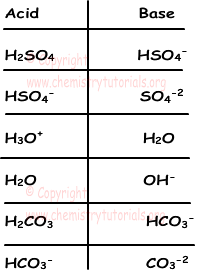Acids and Bases

Acids and Bases

Matters can be classified in many ways. Acids and bases are another way of classification of matters. Most of the reactions taking place in water solutions are in acid or base mediums. Definitions of acids and bases are given by Arrhenius and Bronsted -Lowery. They define acids and bases in different ways. Now we examine these definitions by one one.

1) Definition of Arrhenius :

Arrhenius defines acids as " in water solutions matters that  give H+ ion are called acid". Examples of acids are;

HCl(aq) → H+(aq) + Cl-

HNO3(aq) → H+(aq) + NO3-(aq)

H3PO4(aq) → 3H+(aq) + PO4-3(aq)

H2SO4(aq) → 2H+(aq) + SO4-2(aq)

As you can see from the examples, acids give H+ ion to solution. On the contrary, some of the acids like SO2, P2O5, SO3 do not include H atom, but their solutions with water shows acidic property. Solutions of these matters with water becomes;

SO2(g) + H2O(l) → H2SO3(aq)

CO2(g) + H2O(l) → H2CO3(aq)

P2O5(s) + 3H2O(l) → 2H3PO4(aq)

These non metal oxides are called "anhydrous acids".

Arrhenius defines bases as " in water solutions matters that  give OH- ion are called bases". Examples of bases are;

KOH(s) → K+(aq) + OH-(aq)

Ba(OH)2(s) → Ba+2(aq) + 2OH-(aq)

NaOH(s) → Na+(aq) + OH-(aq)

Ca(OH)2(s) → Ca+2(aq) + 2OH-(aq)

As you can see from the examples, bases give OH- ion to solution. On the contrary, some of the bases like NH3, MgO, CaO do not include OH- ion, but their solutions with water includes OH- ion and they show basic property. Look at these examples given below;

NH3(aq) + H2O(l) → NH4+(aq) + OH-(aq)

K2O(s) + H2O(l) → 2K+(aq) + 2OH-(aq)

MgO(s) + H2O(l) → Mg+2(aq) + 2OH-(aq)

These matters are called "anhydrous bases".

2) Definition of Bronsted-Lowry :

There are some limitations in Arrhenius definition. For example, it can not explain anhydrous acids and bases. Thus, Bronsted-Lowry state another definition for acids and bases. They define acids and bases like;

"Acids are matters that donates H+ and bases are matters that accept H+ ion."

HCl(g) + NH3(g) → NH4+(s) + Cl-(s)

In this reaction, HCl donates H+ ion so it is acid and NH3 accepts H+ ion, it is base.

CO3-2 + H2O → HCO3- + OH-

In this reaction one acid and one base react to give another acid and base. H2O acid donates H+ ion and becomes OH- base, CO3- base accepts H+ ion and becomes HCO3- acid. OH- is the conjugate base of H2O and HCO3- is conjugate acid of CO3-2. List given below shows some common acid and base conjugates;Example: According to Bronsted-Lowry definition, which ones of the following statements are true for following reaction.

HCO3- + HSO4- → H2CO3 + SO4-2

I. Acidic property of HSO4- ion is higher than acidic property of HCO3-

II. SO4-2 ion shows both acidic and basic property.

III. Acidic property of H2CO3 is higher than HSO4- ion.

Solution:

In given reaction, HSO4- and H2CO3 are acid and HCO3- and SO4-2 are base. Since HSO4- ion donate H+ ion to HCO3-, acidic property of it is high. I is true.

Acids should have H+ ion in its structure to become Bronsted-Lowry acid. Since there is no H+ ion in SO4-2, it does not behave like acid. II is false.

Third statement  can be true or false, we can not say it is true by looking at this reaction.

Example: Which ones of the following couples are acid in given reaction?

H2PO4- + HSO3- ↔ HPO4- + H2SO3

I. H2PO4- and H2SO3

II. HSO3- and H2SO3

III. HSO3- and HPO4-

IV. H2PO4- and HPO4-

Solution:

H2PO4- donates H+ and becomes HPO4- so it shows acidic property.

H2SO3 donates H+ and becomes HSO3- so it shows acidic property.

Couple given in I is true.

Acids and Bases Exams and  Problem Solutions

Related